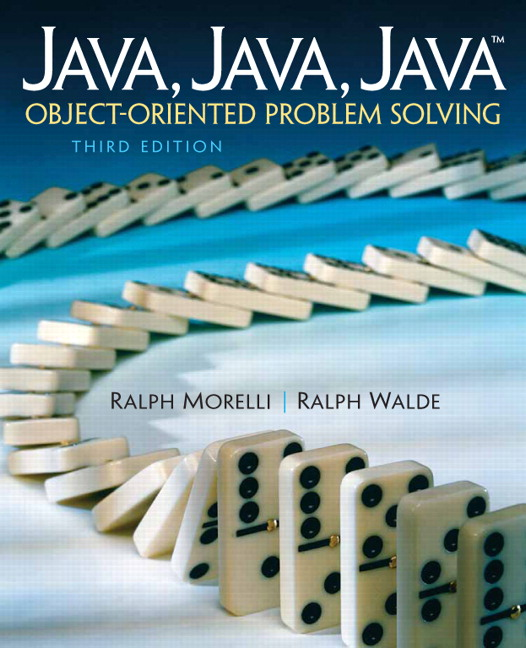# Java, Java, Java: Object-Oriented Problem Solving, 2022E

## Section5.12Chapter Summary

### Subsection5.12.1Technical Terms

 action input-process-output representation binary digit (bit) named constant round off error binary operator operand short-circuit evaluation boundary value operator overloading type conversion cast operation precedence order unary operator class constant promotion Unicode

### Subsection5.12.2Summary of Important Points

• The way we approach a problem can often help or hinder us in our ability to solve it. Choosing an appropriate representation for a problem is often the key to solving it.
• In order to evaluate complex expressions, it is necessary to understand the precedence order and associativity of the operators involved. Parentheses can always be used to override an operator’s built-in precedence.
• Java provides several types of integer data, including the 8-bit byte, 16-bit short, 32-bit int, and 64-bit long types. Unless otherwise specified, integer literals are represented as int data in a Java program.
• Java provides two types of floating-point data, the 32-bit float type and the 64-bit double type. Unless otherwise specified, floating-point literals are represented as double data.
• In general, if a data type uses n bits in its representation, then it can represent $$2^n$$ different values.
• The fact that Java’s primitive types are defined in terms of a specific number of bits is one way that Java promotes platform independence.
• It is necessary to distinguish integer operations from floating-point operations even though the same symbols are used. For example, (7/2) is 3, while (7.0/2) is 3.0.
• In revising a class that is used by other classes it is important to preserve as much of the class’s interface as possible.
• In Java, character data are based on the Unicode character set, which provides $$2^{16}$$ = 65,536 different character codes. To provide backward compatibility with the ASCII code, the first 128 characters are the ASCII coded characters.
• Java operators are evaluated according to the precedence hierarchy shown in the table below. The lower the precedence number, the earlier an operator is evaluated. So the operators at the top of the table are evaluated before operators that occur below them in the table. Operators at the same precedence level are evaluated according to their association, either left to right (L to R) or right to left (R to L).
 Order Operator Operation Association 0 ( ) Parentheses   1 ++ --  Postincrement, postdecrement L to R 1 . dot operator L to R 2 ++ --  Preincrement, predecrement R to L 2 + - unary plus, unary minus R to L 2  !  Boolean NOT R to L 3 (type) new Type cast, object instantiation R to L 4 * / % Multiplication, division, modulus L to R 5 + - Addition, subtraction L to R 5 + String concatenation L to R 6 < > <= >= Relational operators L to R 7 == != Equality operators L to R 8 ^ Boolean XOR L to R 9 && Boolean AND L to R 10 || Boolean OR L to R 11 = += -= *= /= %= assignment operators R to L